# HSSlive: Plus One & Plus Two Notes & Solutions for Kerala State Board

## AP Board Class 7 Maths Chapter 8 Congruency of Triangles InText Questions Textbook Solutions PDF: Download Andhra Pradesh Board STD 7th Maths Chapter 8 Congruency of Triangles InText Questions Book AnswersAP Board Class 7 Maths Chapter 8 Congruency of Triangles InText Questions Textbook Solutions PDF: Download Andhra Pradesh Board STD 7th Maths Chapter 8 Congruency of Triangles InText Questions Book Answers

## Andhra Pradesh State Board Class 7th Maths Chapter 8 Congruency of Triangles InText Questions Books Solutions

 Board AP Board Materials Textbook Solutions/Guide Format DOC/PDF Class 7th Subject Maths Chapters Maths Chapter 8 Congruency of Triangles InText Questions Provider Hsslive

2. Click on the Andhra Pradesh Board Class 7th Maths Chapter 8 Congruency of Triangles InText Questions Answers.
3. Look for your Andhra Pradesh Board STD 7th Maths Chapter 8 Congruency of Triangles InText Questions Textbooks PDF.
4. Now download or read the Andhra Pradesh Board Class 7th Maths Chapter 8 Congruency of Triangles InText Questions Textbook Solutions for PDF Free.

## AP Board Class 7th Maths Chapter 8 Congruency of Triangles InText Questions Textbooks Solutions with Answer PDF Download

Find below the list of all AP Board Class 7th Maths Chapter 8 Congruency of Triangles InText Questions Textbook Solutions for PDF’s for you to download and prepare for the upcoming exams:

Do This

Question 1.
Here are some shapes. See whether all the shapes given in row are congruent to each other or not. You can trace the figures and check. (Page No. 164 )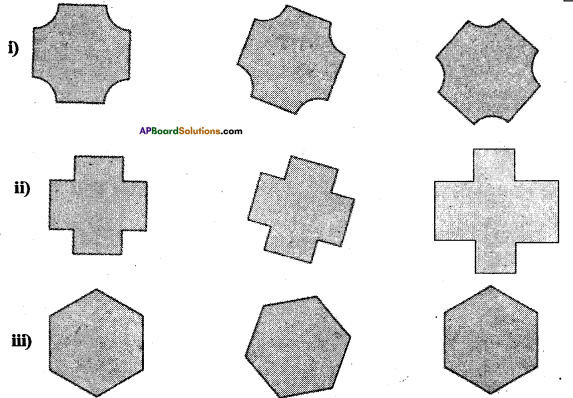Solution:
All are congruent.

Question 2.
Which of the following pairs of figures are congruent ? (Page No. 164 )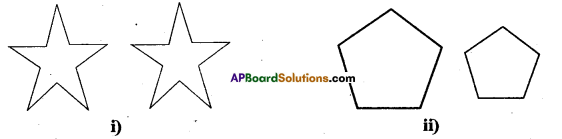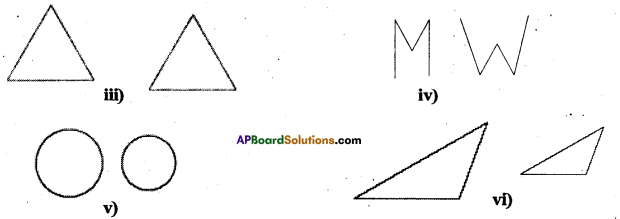Solution:
Both is (i) and (iii) are congruent.

Question 3.
ΔEFG ≅ ΔLMN (Page No. 166)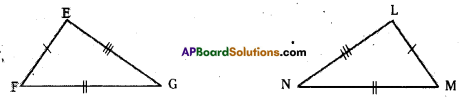Write the corresponding vertices, angles and sides of the two triangles.
Solution:
Corresponding Sides EF = LM; FG = MN; EG = LN
Angles ∠E = ∠L, ∠F = ∠M, ∠G = ∠N
Vertices E = L, F = M, G = N

Question 4.
If ΔABC ≅ ΔDEF, write the parts of AABC that correspond to (Page No. 166)
i) DE
ii) ∠E
iii) DF
iv) EF
v) ∠F
Solution:
i) DE−→−=AB−→−
ii) ∠E = ∠B
iii) DF−→=AC⎯⎯⎯⎯⎯⎯⎯⎯
iv) EF⎯⎯⎯⎯⎯⎯⎯=BC⎯⎯⎯⎯⎯⎯⎯⎯
ii) ∠F = ∠C

Question 5.
Name the congruent triangles in each of the following pairs. Write the statement using ≅. (Page No. 166)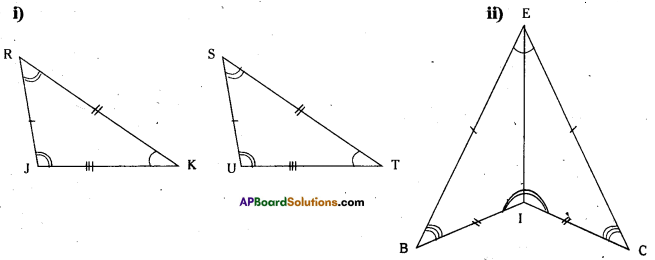Solution:
i) ΔRJK ≅ ΔSUT
ii) ΔBIE ≅ ΔCIE

Question 6.
Name the congruent angles and sides for each pair of congruent triangles. (Page No. 166)
i) ΔCDG ≅ ΔRSW
Solution:
i) ΔTUV ≅ ΔXYZ
Sides: TU = XY
UV = YZ
TV = XZ
Angles :∠T = ∠X,
∠U = ∠Y,
∠V =∠Z

ii) ΔCDG ≅ ΔRSW
Sides : CD = RS
DG = SW
CG = RW
Angles : ∠C = ∠R,
∠D = ∠S,
∠G = ∠W

Try This

Question 1.
Is the following pair of triangles congurent ? Give reason to support your answer. (Page No. 174)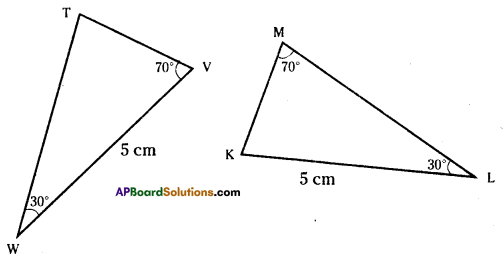Solution:
The triangles are not congruent since the corresponding parts are not equal.

Try This

Question 1.
In the figures given below, measures of some parts of triangles are given. By applying RHS congruence rule state, which pairs of triangle are congruent. In case of congruent tri¬angles, write the result in symbolic form. (Page No. 177)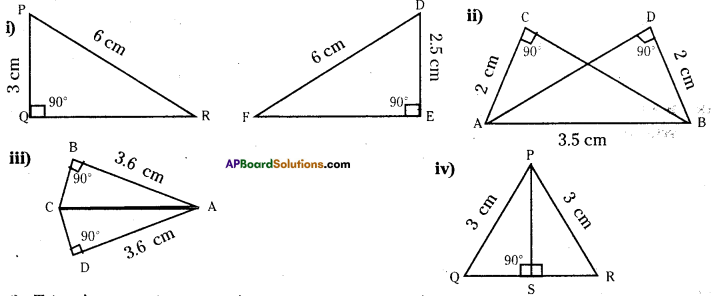Solution:
i) Triangles are not congruent.
ii) ΔABC ≅ ΔBAD or ΔACB ≅ ΔBDA
iv) ΔPQS ≅ ΔPRS

Question 2.
It is to be established by R.H.S congruence rule that ΔABC = ΔRPQ. What additional information is needed, if it is given that ∠B = ∠P = 90° and AB = RP ? (Page No. 177)
Solution:
∠B = ∠P (Right angle)
AB = RP (Side)
So we need AC = RQ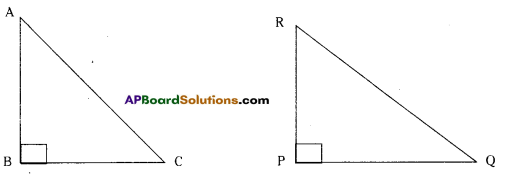Question 3.
In the adjacent figure, BD and CE are altitudes of ΔABC such that BD = CE.
i) State the three pairs of equal parts in ΔCBD andΔBCE. (Page No. 177)
Solution:
∠CDB ≅ ∠BEC (Right angle)
BD = CE (Side)
BC = BC common/hypotenuse

ii) Is ΔCBD ≅ ΔBCE ? Why or why not ?
Solution:
Yes, ΔCBD ≅ ΔBCE by R.H.S congruence.

iii) Is ∠DBC = ∠EBC ? Why or why not ?
Solution:
No, ∠DBC ≠ ∠EBC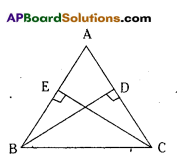Question 4.
ABC is an isosceles triangle with AB = AC and AD is one of its altitudes. Page No. 177
i) State the three pairs of equal parts in ΔADB and ΔADC.
Solution:

Solution:
Yes, by R.H.S congruence

iii) Is ∠B = ∠C ? Why or why not ?
Solution:
Yes by c.p.c.t

iv) Is BD ≅ CD ? Why or why not ?
Solution:
Yes, by c.p.c.t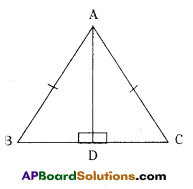## Andhra Pradesh Board Class 7th Maths Chapter 8 Congruency of Triangles InText Questions Textbooks for Exam Preparations

Andhra Pradesh Board Class 7th Maths Chapter 8 Congruency of Triangles InText Questions Textbook Solutions can be of great help in your Andhra Pradesh Board Class 7th Maths Chapter 8 Congruency of Triangles InText Questions exam preparation. The AP Board STD 7th Maths Chapter 8 Congruency of Triangles InText Questions Textbooks study material, used with the English medium textbooks, can help you complete the entire Class 7th Maths Chapter 8 Congruency of Triangles InText Questions Books State Board syllabus with maximum efficiency.

## FAQs Regarding Andhra Pradesh Board Class 7th Maths Chapter 8 Congruency of Triangles InText Questions Textbook Solutions

#### Can we get a Andhra Pradesh State Board Book PDF for all Classes?

Yes you can get Andhra Pradesh Board Text Book PDF for all classes using the links provided in the above article.

## Important Terms

Andhra Pradesh Board Class 7th Maths Chapter 8 Congruency of Triangles InText Questions, AP Board Class 7th Maths Chapter 8 Congruency of Triangles InText Questions Textbooks, Andhra Pradesh State Board Class 7th Maths Chapter 8 Congruency of Triangles InText Questions, Andhra Pradesh State Board Class 7th Maths Chapter 8 Congruency of Triangles InText Questions Textbook solutions, AP Board Class 7th Maths Chapter 8 Congruency of Triangles InText Questions Textbooks Solutions, Andhra Pradesh Board STD 7th Maths Chapter 8 Congruency of Triangles InText Questions, AP Board STD 7th Maths Chapter 8 Congruency of Triangles InText Questions Textbooks, Andhra Pradesh State Board STD 7th Maths Chapter 8 Congruency of Triangles InText Questions, Andhra Pradesh State Board STD 7th Maths Chapter 8 Congruency of Triangles InText Questions Textbook solutions, AP Board STD 7th Maths Chapter 8 Congruency of Triangles InText Questions Textbooks Solutions,
Share: